# Batch Norm and Transfer Learning — whats going on?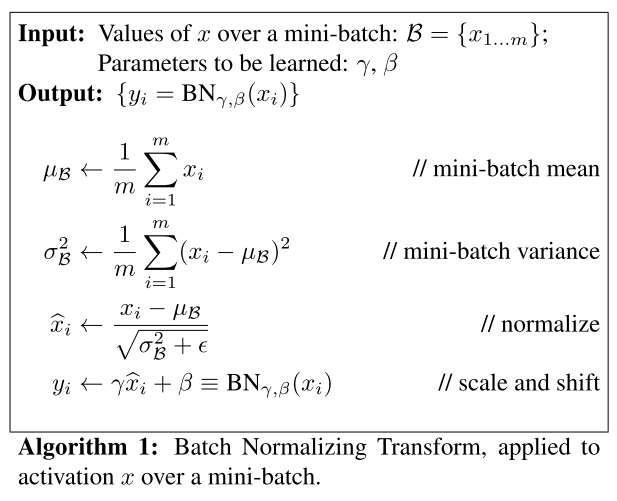after Ioffe and Szegedy (2015)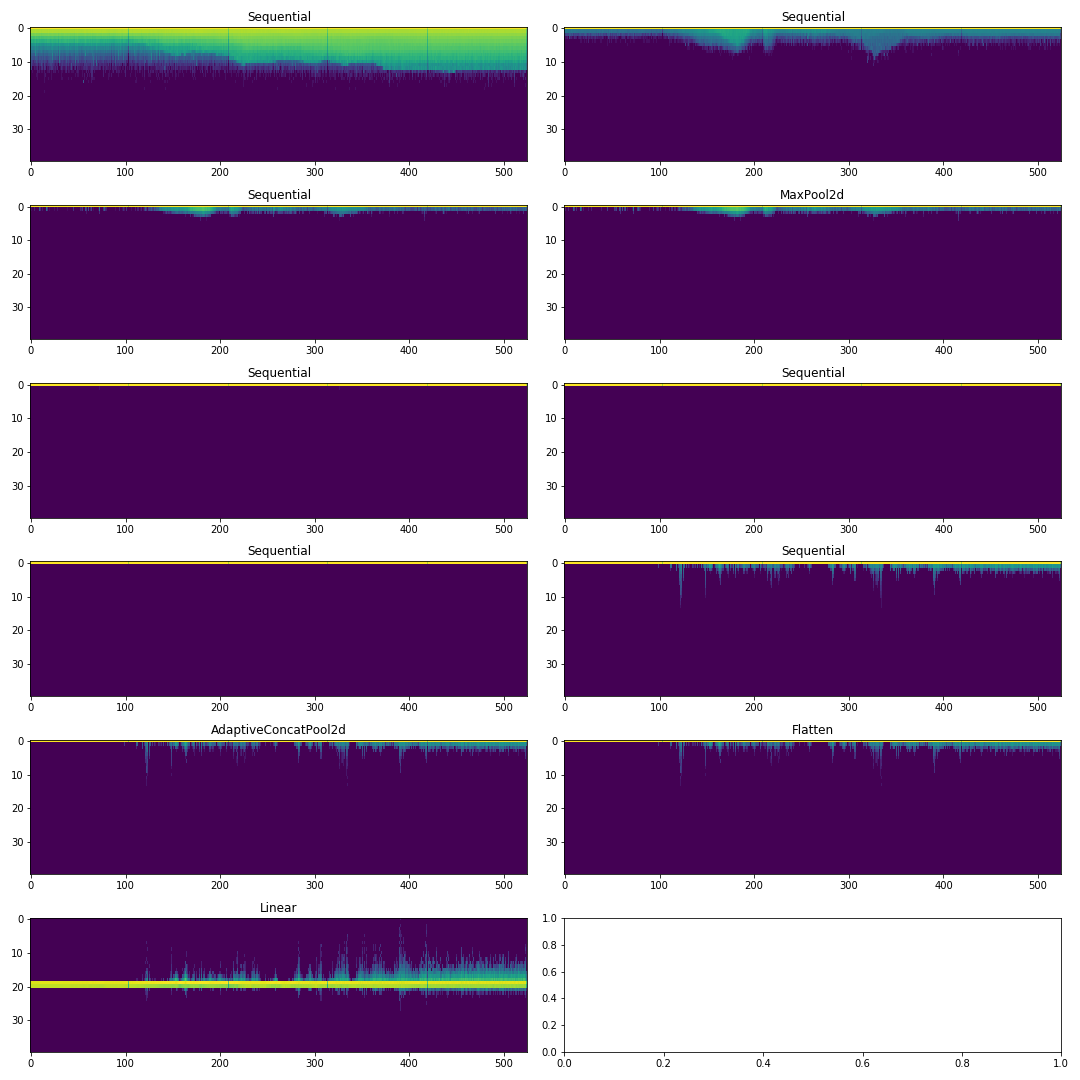Fig. 1. weights for each minibatch (x) plotted as histogram with 40 bins (y).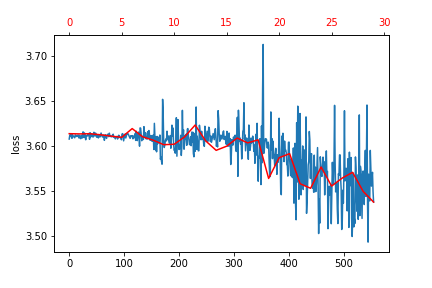Fig. 2. loss vs minibatch for training (blue) and validation (red) data for ‘Naive model’ without batch norm.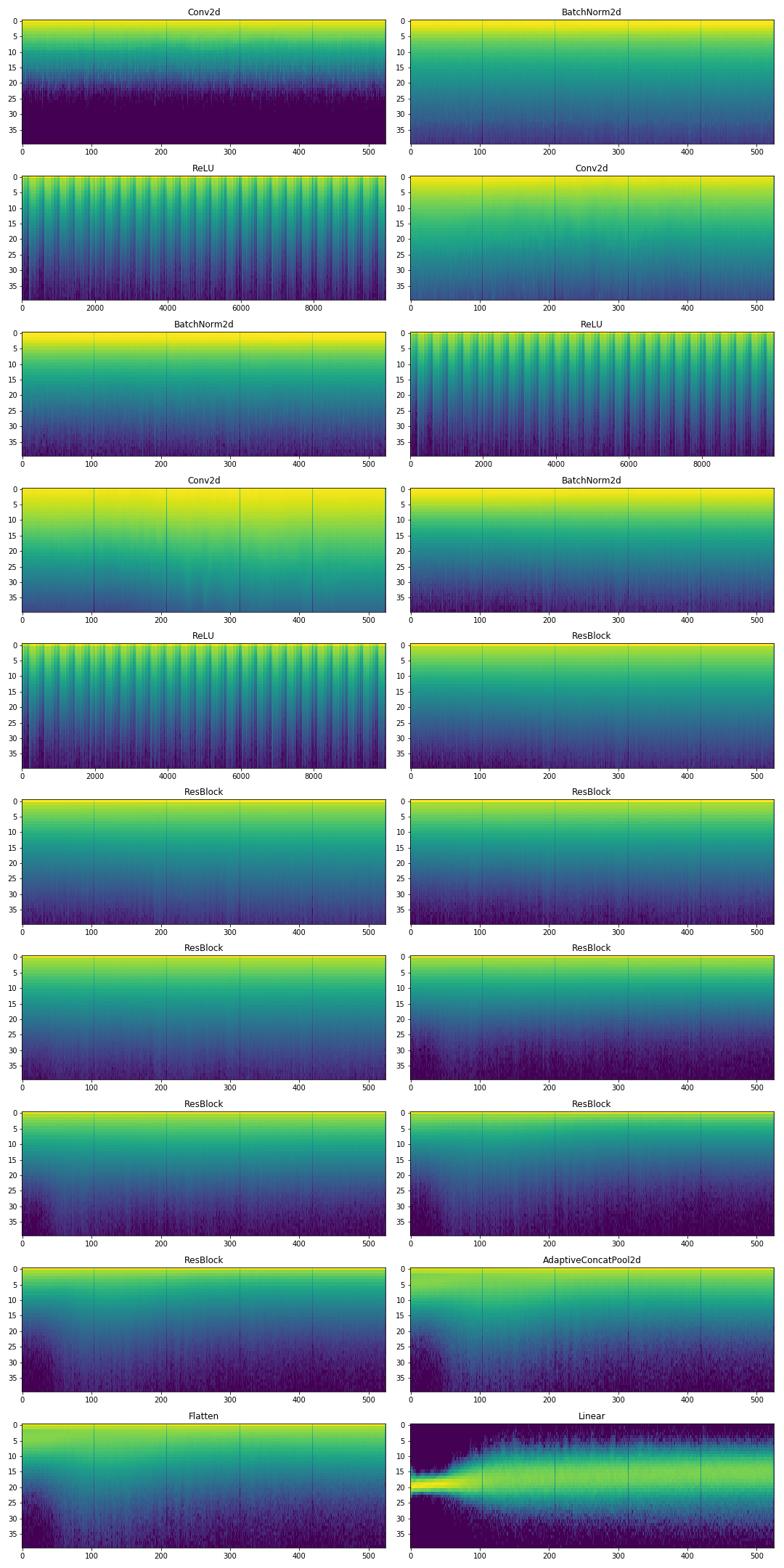Fig. 3. histograms for ‘Naive model’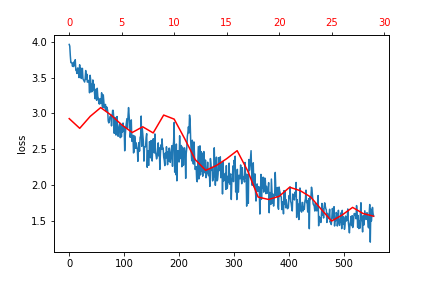Fig. 4. loss vs minibatch for training (blue) and validation (red) data for ‘Naive model’ with batch norm.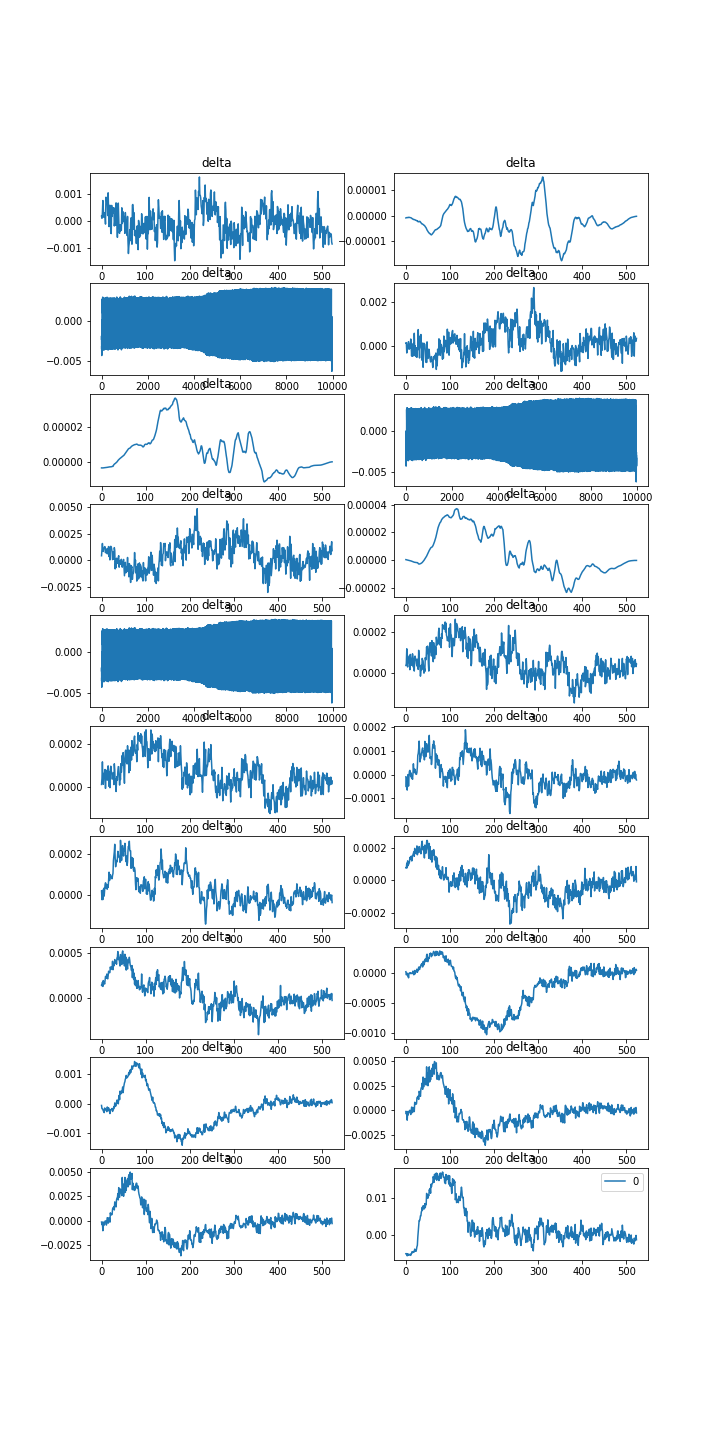Fig. 5. change in variance of weights per batch for each layer in the model. Batch Norm has a clear smoothing effect.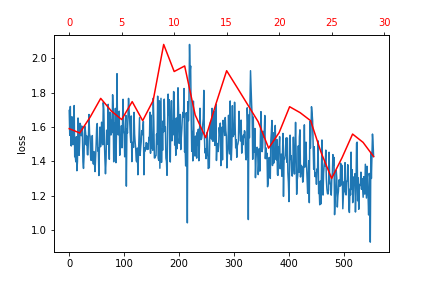Fig. 6. loss for Unfreeze model.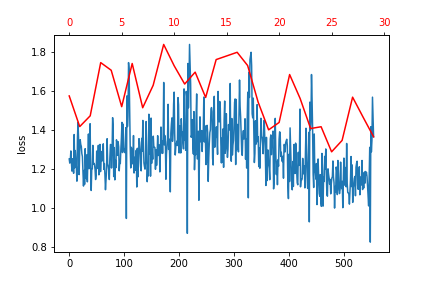Fig. 7. loss for Unfreeze non BN model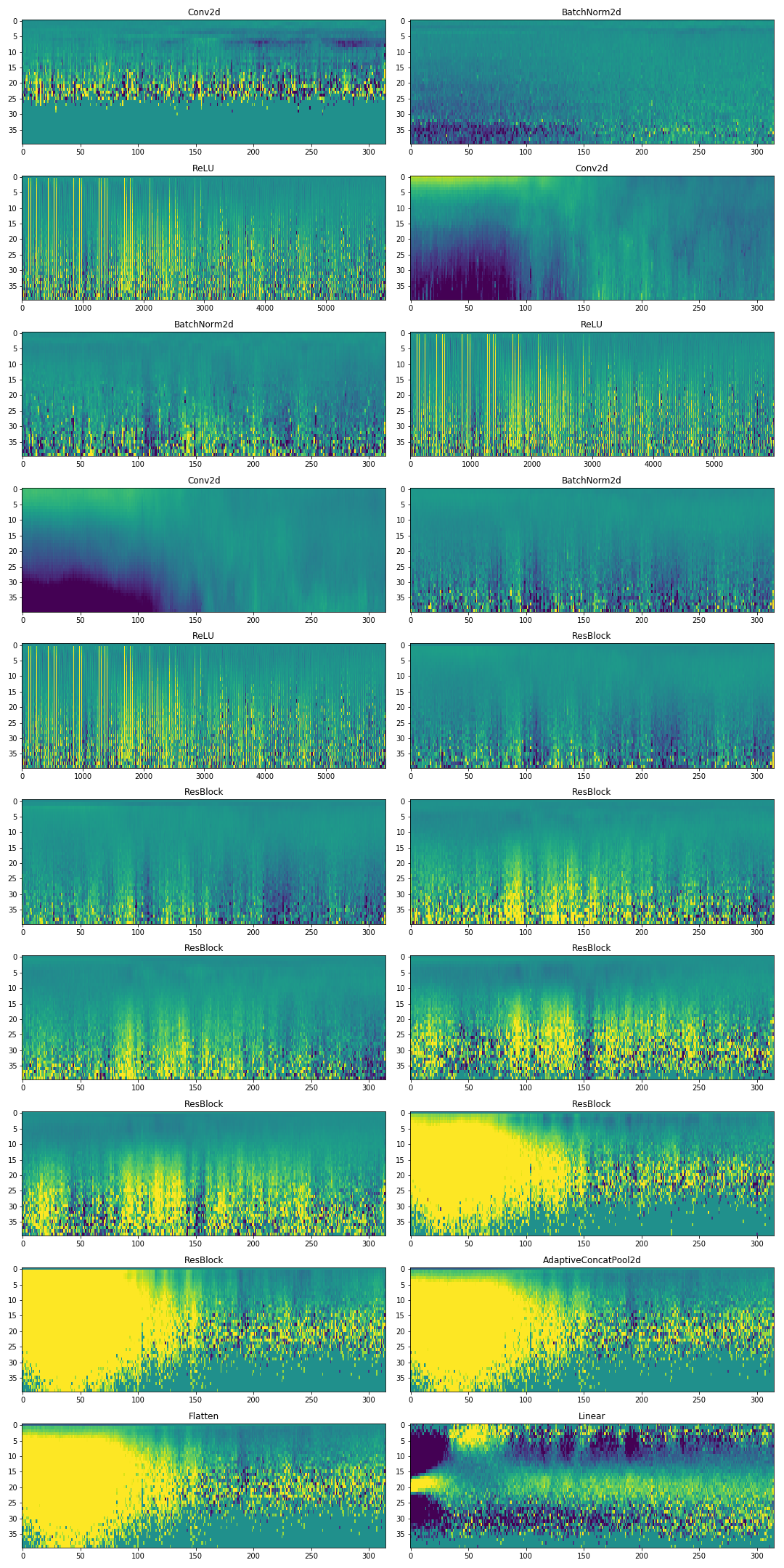Fig. 8. Difference in weights for 3 epochs of Free model w.r.t Unfreeze model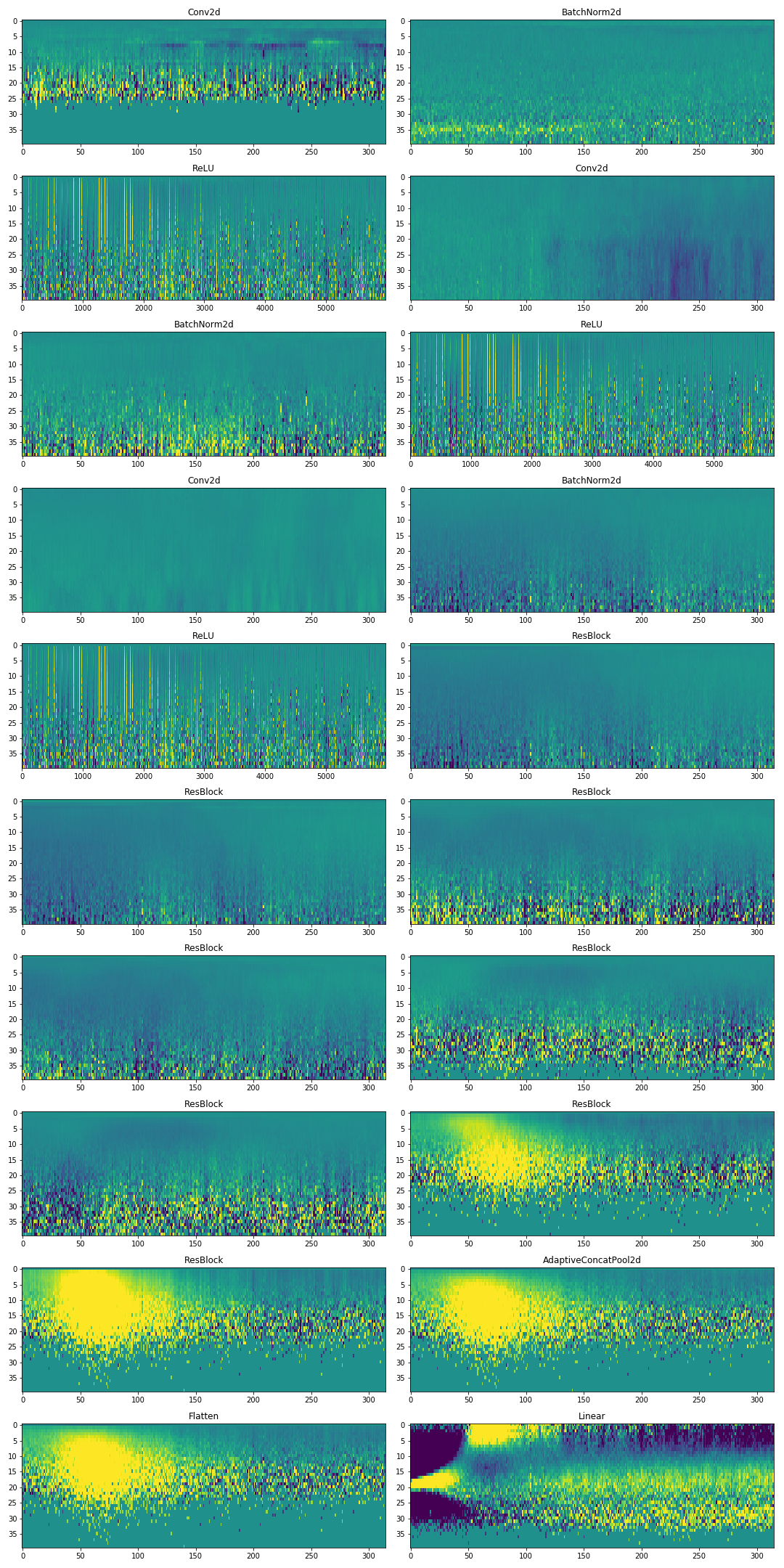Fig. 9. Difference in weights for 3 epochs of Freeze non BN model w.r.t Unfreeze non BN model.

--

--